Anúncio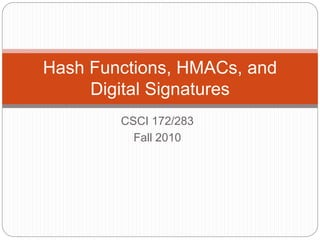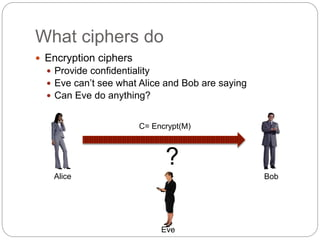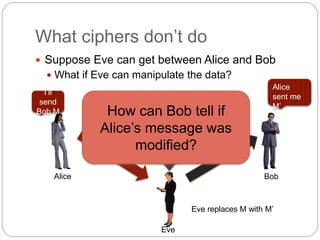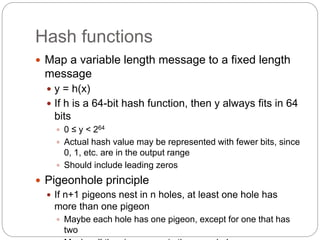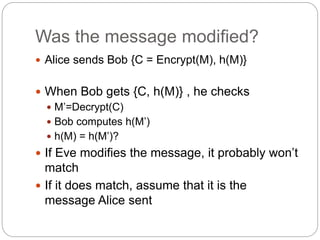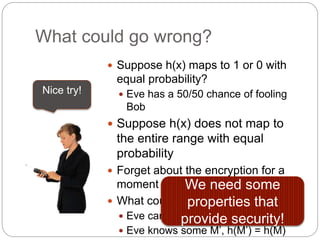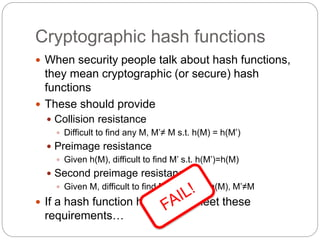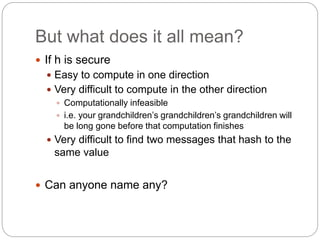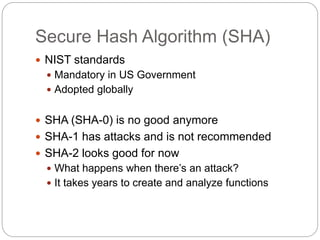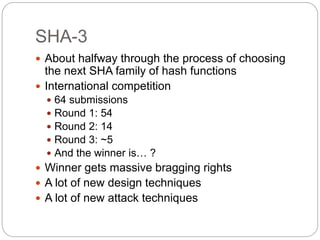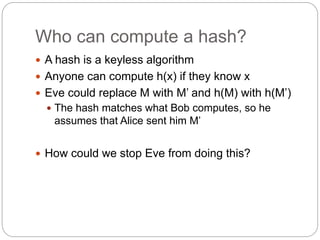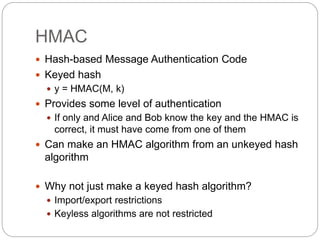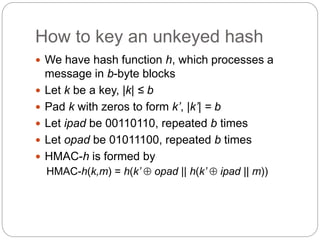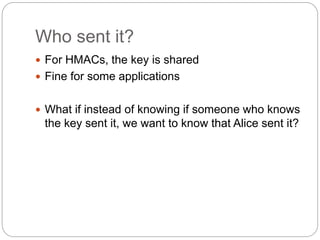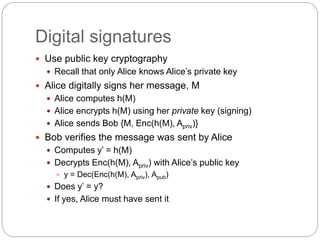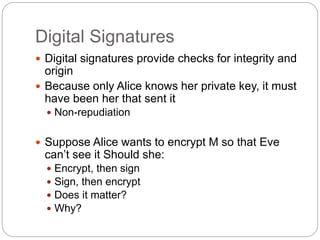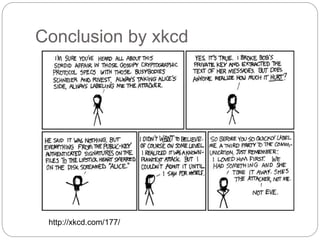1 de 17
Anúncio

### CS283_hash.ppt

1. CSCI 172/283 Fall 2010 Hash Functions, HMACs, and Digital Signatures
2. What ciphers do  Encryption ciphers  Provide confidentiality  Eve can’t see what Alice and Bob are saying  Can Eve do anything? Alice Bob Eve ? C= Encrypt(M)
3. What ciphers don’t do  Suppose Eve can get between Alice and Bob  What if Eve can manipulate the data? Alice Bob Eve M M’ I’ll send Bob M Alice sent me M’ Now for a few changes Eve replaces M with M’ How can Bob tell if Alice’s message was modified?
4. Hash functions  Map a variable length message to a fixed length message  y = h(x)  If h is a 64-bit hash function, then y always fits in 64 bits  0 ≤ y < 264  Actual hash value may be represented with fewer bits, since 0, 1, etc. are in the output range  Should include leading zeros  Pigeonhole principle  If n+1 pigeons nest in n holes, at least one hole has more than one pigeon  Maybe each hole has one pigeon, except for one that has two
5. Was the message modified?  Alice sends Bob {C = Encrypt(M), h(M)}  When Bob gets {C, h(M)} , he checks  M’=Decrypt(C)  Bob computes h(M’)  h(M) = h(M’)?  If Eve modifies the message, it probably won’t match  If it does match, assume that it is the message Alice sent
6. What could go wrong?  Suppose h(x) maps to 1 or 0 with equal probability?  Eve has a 50/50 chance of fooling Bob  Suppose h(x) does not map to the entire range with equal probability  Forget about the encryption for a moment  What could Eve do? Suppose:  Eve can calculate f(h(M)) = M  Eve knows some M’, h(M’) = h(M) Nice try! We need some properties that provide security!
7. Cryptographic hash functions  When security people talk about hash functions, they mean cryptographic (or secure) hash functions  These should provide  Collision resistance  Difficult to find any M, M’≠ M s.t. h(M) = h(M’)  Preimage resistance  Given h(M), difficult to find M’ s.t. h(M’)=h(M)  Second preimage resistance  Given M, difficult to find M’ s.t. h(M’)=h(M), M’≠M  If a hash function h does not meet these requirements…
8. But what does it all mean?  If h is secure  Easy to compute in one direction  Very difficult to compute in the other direction  Computationally infeasible  i.e. your grandchildren’s grandchildren’s grandchildren will be long gone before that computation finishes  Very difficult to find two messages that hash to the same value  Can anyone name any?
9. Secure Hash Algorithm (SHA)  NIST standards  Mandatory in US Government  Adopted globally  SHA (SHA-0) is no good anymore  SHA-1 has attacks and is not recommended  SHA-2 looks good for now  What happens when there’s an attack?  It takes years to create and analyze functions
10. SHA-3  About halfway through the process of choosing the next SHA family of hash functions  International competition  64 submissions  Round 1: 54  Round 2: 14  Round 3: ~5  And the winner is… ?  Winner gets massive bragging rights  A lot of new design techniques  A lot of new attack techniques
11. Who can compute a hash?  A hash is a keyless algorithm  Anyone can compute h(x) if they know x  Eve could replace M with M’ and h(M) with h(M’)  The hash matches what Bob computes, so he assumes that Alice sent him M’  How could we stop Eve from doing this?
12. HMAC  Hash-based Message Authentication Code  Keyed hash  y = HMAC(M, k)  Provides some level of authentication  If only and Alice and Bob know the key and the HMAC is correct, it must have come from one of them  Can make an HMAC algorithm from an unkeyed hash algorithm  Why not just make a keyed hash algorithm?  Import/export restrictions  Keyless algorithms are not restricted
13. How to key an unkeyed hash  We have hash function h, which processes a message in b-byte blocks  Let k be a key, |k| ≤ b  Pad k with zeros to form k’, |k’| = b  Let ipad be 00110110, repeated b times  Let opad be 01011100, repeated b times  HMAC-h is formed by HMAC-h(k,m) = h(k’  opad || h(k’  ipad || m))
14. Who sent it?  For HMACs, the key is shared  Fine for some applications  What if instead of knowing if someone who knows the key sent it, we want to know that Alice sent it?
15. Digital signatures  Use public key cryptography  Recall that only Alice knows Alice’s private key  Alice digitally signs her message, M  Alice computes h(M)  Alice encrypts h(M) using her private key (signing)  Alice sends Bob {M, Enc(h(M), Apriv)}  Bob verifies the message was sent by Alice  Computes y’ = h(M)  Decrypts Enc(h(M), Apriv) with Alice’s public key  y = Dec(Enc(h(M), Apriv), Apub)  Does y’ = y?  If yes, Alice must have sent it
16. Digital Signatures  Digital signatures provide checks for integrity and origin  Because only Alice knows her private key, it must have been her that sent it  Non-repudiation  Suppose Alice wants to encrypt M so that Eve can’t see it Should she:  Encrypt, then sign  Sign, then encrypt  Does it matter?  Why?
17. Conclusion by xkcd http://xkcd.com/177/
Anúncio